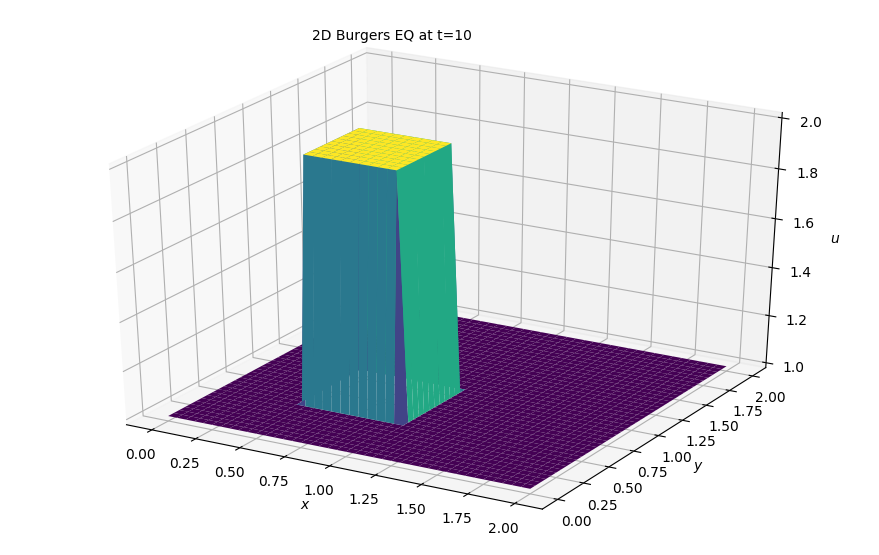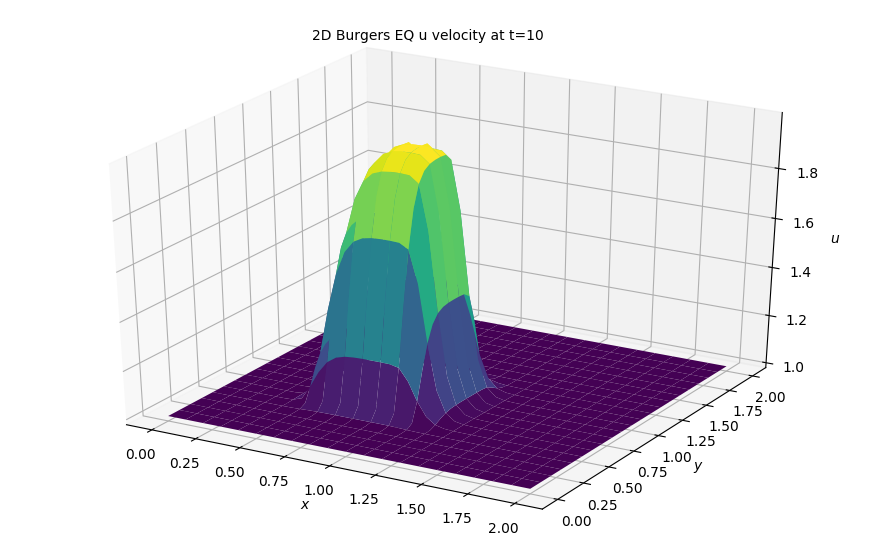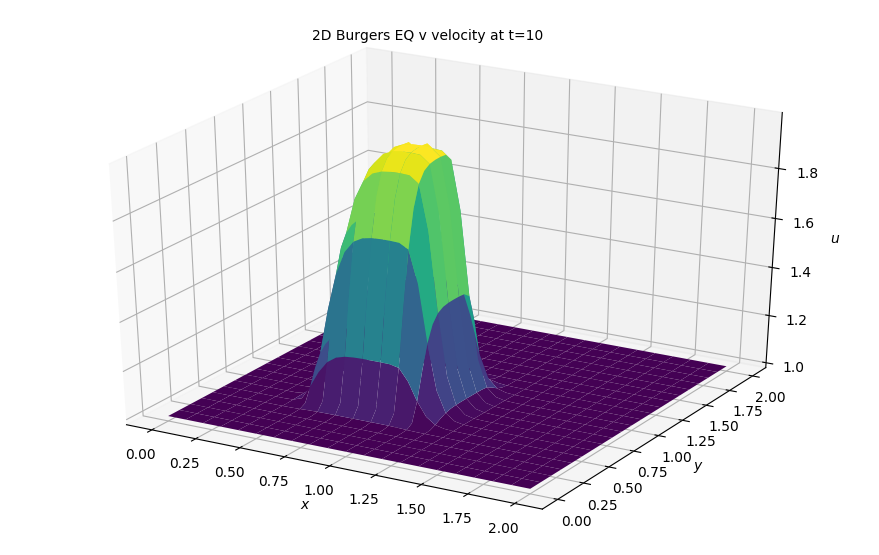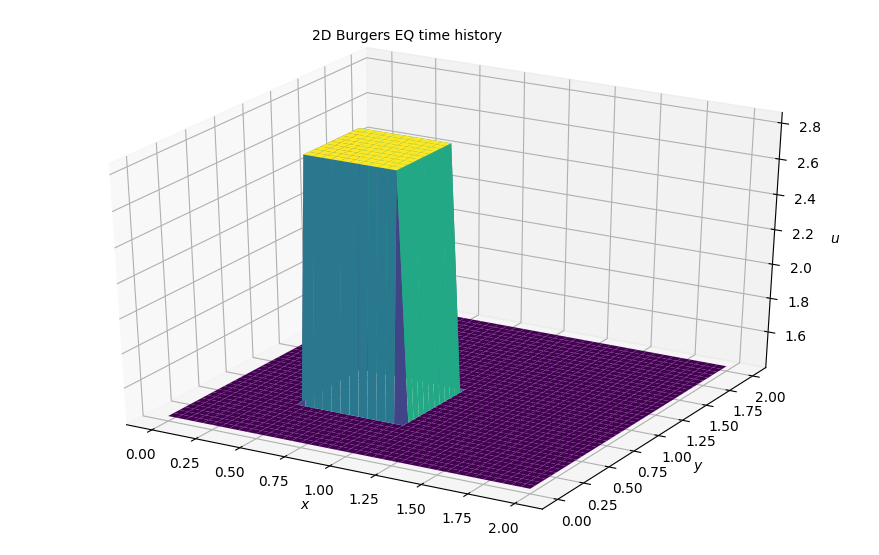# Step 8: 2D Burgers Equation¶

This is a major milestone in our quest towards solving the Navier Stokes equations in 2D in python. The burger's equation is great to learn about CFD and fluid dynamics in general because it contains the full convective nonlinearity of the flow equations and at the same time has many known analytic solutions. An interesting thing about it is that it can generate discontinuous solutiosn from an initial condition that is smooth, in other words it can develop shocks. We wnat to see this occur in two dimensions. Let's start with our set of coupled PDEs:

$$\frac{\partial u}{\partial t} + u \frac{\partial u}{\partial x} + v \frac{\partial u}{\partial y} = \nu \left (\frac{\partial^2 u}{\partial x^2} + \frac{\partial^2 u}{\partial y^2} \right )$$$$\frac{\partial v}{\partial t} + u \frac{\partial v}{\partial x} + v \frac{\partial v}{\partial y} = \nu \left (\frac{\partial^2 v}{\partial x^2} + \frac{\partial^2 v}{\partial y^2} \right )$$

## Discretization¶

We've built a pretty good amount of experience in discretizing these terms. Here are the results:

$$\frac{u_{i,j}^{n+1} - u_{i,j}^n}{\Delta t} + u_{i,j}^n \frac{u_{i,j}^n-u_{i-1,j}^n}{\Delta x} + v_{i,j}^n \frac{u_{i,j}^n - u_{i,j-1}^n}{\Delta y} =$$

$$\qquad \nu \bigg( \frac{u_{i+1,j}^n - 2u_{i,j}^n+u_{i-1,j}^n}{\Delta x^2} + \frac{u_{i,j+1}^n - 2u_{i,j}^n + u_{i,j-1}^n}{\Delta y^2} \bigg)$$

$$\frac{v_{i,j}^{n+1} - v_{i,j}^n}{\Delta t} + u_{i,j}^n \frac{v_{i,j}^n-v_{i-1,j}^n}{\Delta x} + v_{i,j}^n \frac{v_{i,j}^n - v_{i,j-1}^n}{\Delta y} =$$$$\nu \bigg( \frac{v_{i+1,j}^n - 2v_{i,j}^n+v_{i-1,j}^n}{\Delta x^2} + \frac{v_{i,j+1}^n - 2v_{i,j}^n + v_{i,j-1}^n}{\Delta y^2} \bigg)$$

As always, we solve for our only unknown $u^{n+1}_{i,j}$ and $v^{n+1}_{i,j}$ and iterate through the equation that follows:

$$u_{i,j}^{n+1} = u_{i,j}^n - \frac{\Delta t}{\Delta x} u_{i,j}^n (u_{i,j}^n - u_{i-1,j}^n) - \frac{\Delta t}{\Delta y} v_{i,j}^n (u_{i,j}^n - u_{i,j-1}^n)+$$ $$\frac{\nu \Delta t}{\Delta x^2}(u_{i+1,j}^n-2u_{i,j}^n+u_{i-1,j}^n) + \frac{\nu \Delta t}{\Delta y^2} (u_{i,j+1}^n - 2u_{i,j}^n + u_{i,j+1}^n)$$

$$v_{i,j}^{n+1} = v_{i,j}^n - \frac{\Delta t}{\Delta x} u_{i,j}^n (v_{i,j}^n - v_{i-1,j}^n) - \frac{\Delta t}{\Delta y} v_{i,j}^n (v_{i,j}^n - v_{i,j-1}^n)+$$ $$\frac{\nu \Delta t}{\Delta x^2}(v_{i+1,j}^n-2v_{i,j}^n+v_{i-1,j}^n) + \frac{\nu \Delta t}{\Delta y^2} (v_{i,j+1}^n - 2v_{i,j}^n + v_{i,j+1}^n)$$

## Initial and Boundary Conditions¶

We will solve the equation with the same initial conditions:

$$u,v = \begin{cases} \begin{matrix} 2 & \text{for } x,y \in (0.5,1) \times (0.5,1) \cr 1 & \text{everywhere else} \end{matrix}\end{cases}$$

And boundary conditions:

$$u = 1, v=1 \ \text{for} \begin{cases} \begin{matrix} x = 0,2 \cr y = 0,2 \end{matrix} \end{cases}$$

The boundary conditions set u and v equal to 1 along boundaries of the grid:

## Libraries and Variable declarations¶

### Lib import¶

In :
# Adding inline command to make plots appear under comments
import numpy as np
import matplotlib.pyplot as plt
from matplotlib import cm
from mpl_toolkits.mplot3d import Axes3D
%matplotlib notebook


### Variable Declarations¶

In :
grid_length_x = 2
grid_length_y = 2
grid_points_x = 41
grid_points_y = 41
nt = 240
c = 1
nu = .01
dx = grid_length_x / (grid_points_x - 1)
dy = grid_length_y / (grid_points_y - 1)
sigma = .0009
dt = sigma * dx * dy / nu

x = np.linspace(0, grid_length_x, grid_points_x)
y = np.linspace(0, grid_length_y, grid_points_y)

u = np.ones((grid_points_x, grid_points_y))
v = np.ones((grid_points_x, grid_points_y))
un = np.ones((grid_points_x, grid_points_y))
vn = np.ones((grid_points_x, grid_points_y))

#Initiallizing the array containing the shape of our initial conditions
u[int(.5 / dy):int(1 / dy + 1),int(.5 / dx):int(1 / dx + 1)] = 2
v[int(.5 / dy):int(1 / dy + 1),int(.5 / dx):int(1 / dx + 1)] = 2


### Plotting initial conditions¶

In :
fig = plt.figure(figsize=(11,7), dpi= 100)
ax = fig.gca(projection='3d')
X,Y = np.meshgrid(x,y)

surf = ax.plot_surface(X,Y, u[:], cmap=cm.viridis, rstride=1, cstride=1)
ax.set_xlabel('$x$')
ax.set_zlabel('$u$')
ax.set_ylabel('$y$')
ax.text2D(0.35, 0.95, "2D Burgers EQ at t=10", transform=ax.transAxes);## Solving in 2D¶

In :
for n in range(nt): ##loop across number of time steps
un = u.copy()
vn = v.copy()

u[1:-1, 1:-1] = (un[1:-1, 1:-1] -
dt / dx * un[1:-1, 1:-1] *
(un[1:-1, 1:-1] - un[1:-1, 0:-2]) -
dt / dy * vn[1:-1, 1:-1] *
(un[1:-1, 1:-1] - un[0:-2, 1:-1]) +
nu * dt / dx**2 *
(un[1:-1,2:] - 2 * un[1:-1, 1:-1] + un[1:-1, 0:-2]) +
nu * dt / dy**2 *
(un[2:, 1:-1] - 2 * un[1:-1, 1:-1] + un[0:-2, 1:-1]))

v[1:-1, 1:-1] = (vn[1:-1, 1:-1] -
dt / dx * un[1:-1, 1:-1] *
(vn[1:-1, 1:-1] - vn[1:-1, 0:-2]) -
dt / dy * vn[1:-1, 1:-1] *
(vn[1:-1, 1:-1] - vn[0:-2, 1:-1]) +
nu * dt / dx**2 *
(vn[1:-1, 2:] - 2 * vn[1:-1, 1:-1] + vn[1:-1, 0:-2]) +
nu * dt / dy**2 *
(vn[2:, 1:-1] - 2 * vn[1:-1, 1:-1] + vn[0:-2, 1:-1]))

u[0, :] = 1
u[-1, :] = 1
u[:, 0] = 1
u[:, -1] = 1

v[0, :] = 1
v[-1, :] = 1
v[:, 0] = 1
v[:, -1] = 1

In :
fig = plt.figure(figsize=(11,7), dpi= 100)
ax = fig.gca(projection='3d')
X,Y = np.meshgrid(x,y)

surf = ax.plot_surface(X,Y, u[:], cmap=cm.viridis, rstride=2, cstride=2)
ax.set_xlabel('$x$')
ax.set_zlabel('$u$')
ax.set_ylabel('$y$')
ax.text2D(0.35, 0.95, "2D Burgers EQ u velocity at t=10", transform=ax.transAxes);In :
fig = plt.figure(figsize=(11,7), dpi= 100)
ax = fig.gca(projection='3d')
X,Y = np.meshgrid(x,y)

surf = ax.plot_surface(X,Y, v[:], cmap=cm.viridis, rstride=2, cstride=2)
ax.set_xlabel('$x$')
ax.set_zlabel('$u$')
ax.set_ylabel('$y$')
ax.text2D(0.35, 0.95, "2D Burgers EQ v velocity at t=10", transform=ax.transAxes);### Results¶

As expected, this looks pretty similar to the combination of the convection and diffussion terms.

## Animating the simulation¶

In :
#Imports for animation and display within a jupyter notebook
from matplotlib import animation, rc
from IPython.display import HTML

#Resetting the U wave back to initial conditions
u = np.ones((grid_points_x, grid_points_y))
u[int(.5 / dy):int(1 / dy + 1), int(.5 / dx):int(1 / dx + 1)] = 2

v = np.ones((grid_points_x, grid_points_y))
v[int(.5 / dy):int(1 / dy + 1), int(.5 / dx):int(1 / dx + 1)] = 2
#Generating the figure that will contain the animation
fig = plt.figure(figsize=(11,7), dpi=100)
ax = fig.gca(projection='3d')

surf = ax.plot_surface(X, Y, np.sqrt(u**2 + v**2) , cmap=cm.viridis)
ax.set_xlabel('$x$')
ax.set_zlabel('$u$')
ax.set_ylabel('$y$')
ax.text2D(0.35, 0.95, "2D Burgers EQ time history", transform=ax.transAxes);In :
#Initialization function for funcanimation
def init():
ax.clear()
surf = ax.plot_surface(X, Y, u , cmap=cm.viridis)
return surf

In :
#Main animation function, each frame represents a time step in our calculation
def animate(j):
un = u.copy()
vn = v.copy()

u[1:-1, 1:-1] = (un[1:-1, 1:-1] -
dt / dx * un[1:-1, 1:-1] *
(un[1:-1, 1:-1] - un[1:-1, 0:-2]) -
dt / dy * vn[1:-1, 1:-1] *
(un[1:-1, 1:-1] - un[0:-2, 1:-1]) +
nu * dt / dx**2 *
(un[1:-1,2:] - 2 * un[1:-1, 1:-1] + un[1:-1, 0:-2]) +
nu * dt / dy**2 *
(un[2:, 1:-1] - 2 * un[1:-1, 1:-1] + un[0:-2, 1:-1]))

v[1:-1, 1:-1] = (vn[1:-1, 1:-1] -
dt / dx * un[1:-1, 1:-1] *
(vn[1:-1, 1:-1] - vn[1:-1, 0:-2]) -
dt / dy * vn[1:-1, 1:-1] *
(vn[1:-1, 1:-1] - vn[0:-2, 1:-1]) +
nu * dt / dx**2 *
(vn[1:-1, 2:] - 2 * vn[1:-1, 1:-1] + vn[1:-1, 0:-2]) +
nu * dt / dy**2 *
(vn[2:, 1:-1] - 2 * vn[1:-1, 1:-1] + vn[0:-2, 1:-1]))

u[0, :] = 1
u[-1, :] = 1
u[:, 0] = 1
u[:, -1] = 1

v[0, :] = 1
v[-1, :] = 1
v[:, 0] = 1
v[:, -1] = 1

ax.clear()
surf = ax.plot_surface(X, Y,u,rstride=1, cstride=1, cmap=cm.viridis, linewidth= 0, antialiased=True)
ax.set_zlim(1, 2.5)
ax.set_xlabel('$x$')
ax.set_zlabel('$u$')
ax.set_ylabel('$y$')
ax.text2D(0.35, 0.95, "2D Burgers EQ v velocity at t=10", transform=ax.transAxes);
return surf

In :
anim = animation.FuncAnimation(fig, animate, init_func=init,
frames=nt, interval=20)
HTML(anim.to_jshtml())

Animation size has reached 21141150 bytes, exceeding the limit of 20971520.0. If you're sure you want a larger animation embedded, set the animation.embed_limit rc parameter to a larger value (in MB). This and further frames will be dropped.

Out:

Once Loop Reflect